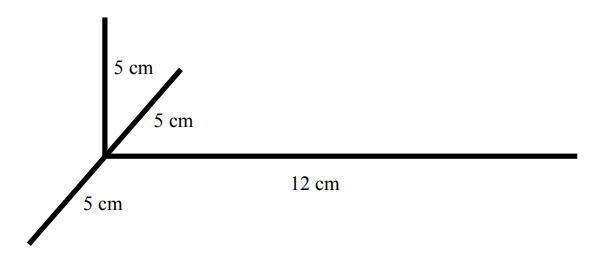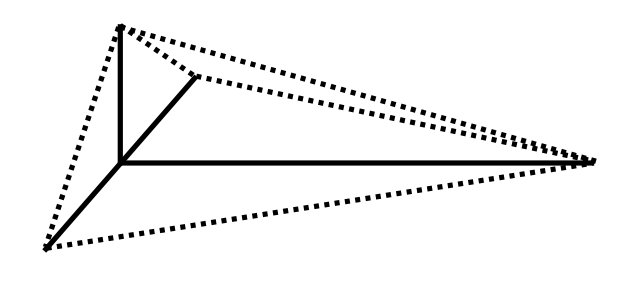Subject:
Mathematics

Topics: Right trianglesTrigonometric Ratios
Common Core State Standard: 8.G.7,
Concepts:

• Right triangle
• Trigonometric ratios (optional)

Knowledge and Skills:

• Can use the Pythagorean Theorem to find the length of the third side of a right triangle, given the lengths of two other sides.
• (Optional): Can find the measurements of all angles in a right triangle, given the lengths of all sides.

Materials:

(for each team)
• Ten 8-inch hollow plastic coffee stirrers
• Fifteen 1-inch lengths of twist-tie material (of a size that fits snugly inside the coffee stirrers)
• One pair of scissors

*It is important to have the right size of coffee stirrers and twist-tie material; in order to assemble the shape, students will need to insert either one or two connecting pieces (twist-ties) into the ends of the coffee stirrer pieces, and either way the fit should be snug enough to hold. Be sure to try this yourself first so you can help as needed.

Lesson:

Procedure: Prepare the Futures Channel movie, “Inventing Toys,” for presentation. Tell students that as they watch the movie, you want them to think about this question (which should be posted):

Why would a toy designer need to know geometry?

At the end of the movie, ask students to work in teams of two or three for a few minutes to list answers to this question. Discuss a few answers.

Distribute the handout to the teams; ask them to read through it, and discuss the situation to ensure that all teams understand the problem they are being asked to solve. Then distribute the materials

Student teams should first perform the calculations, with these steps:

1) Identify the right triangles involved (three right triangles with legs of 5 and 12 centimeters, two right triangles with legs of 5 and 5 centimeters).

2) Use the Pythagorean Theorem to determine the length of the hypotenuses (13 cm and 7.07 cm, respectively).

Students should then cut appropriate lengths of the coffee stirrers and assemble the shapes to verify their calculations. As an optional extension or an alternative activity, you may ask students to find the measures of all angles involved, using what they know of the sides and an understanding of trigonometric ratios.

As another option or extra-credit activity, you may wish to ask student teams to create different basic units of construction (involving right triangles) and thereby demonstrate their ability to apply the Pythagorean Theorem, the definitions of trigonometric ratios, or both.

### Triangular Toys

The toy designers at “Out of This World Toys, Inc.” are designing a new construction set, to be used for building space stations, space vehicles and lunar and Martian habitats. The frame of the basic construction unit looks like this:In order to make the frame stronger, the designers want to add five more pieces, shown here as dotted lines:Can you find the length of each of the five additional pieces? You will need to show your calculations, and make a model to show that your calculations are correct.